Question

Describe the mass, radius, and thus density of a typical white dwarf star. How does this compare to the density of water? What kind of pressure is used by a white dwarf to oppose its strong gravity? How does a white dwarf’s radius depend on its mass, and how does this set the maximum mass of a white dwarf?

The mass of white-dwarf has a limiting mass of 1.4 solar masses (Chandrashekar limit) with radius in the order of about 2% of solar radius. The density of the star is in order of 10^5 to 10^7 kg/m^3. This is around 2-4 orders of higher magnitude as compared to water. The electron degeneracy pressure is used to oppose the strong gravity, It is caused due to the result of Paulli's exclusion principle. The radius is inversely proportional to cube root of mass.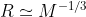The chandrashekar limit gives the maximum mass of white dwarf as 1.4 solar masses.

#### Earn Coins

Coins can be redeemed for fabulous gifts.

Similar Homework Help Questions
• ### If a neutron star and a white dwarf has a total mass of 1M. If the white dwarf has a radius of 9 x 106 m and the neutron star has a radius of 11 km. i) Compare the surface gravity of both stars. ii) W...

If a neutron star and a white dwarf has a total mass of 1M. If the white dwarf has a radius of 9 x 106 m and the neutron star has a radius of 11 km. i) Compare the surface gravity of both stars. ii) What is the density of the neutron star? iii) Assuming the neutron star is entirely made up of neutrons, and that the interparticle separation of a gas of density n is l = n-1/3. How...

• ### Find the average density of a white dwarf star

Find the average density of a white dwarf star if it has a mass equal to that of the sun (2.0 * 10^30 kg) and a radius equal to that of the Earth (6.4 * 10^6 m).

• ### 3.(a) Using for kinetic and gravitational energies of the white dwarf star simplified ex pression...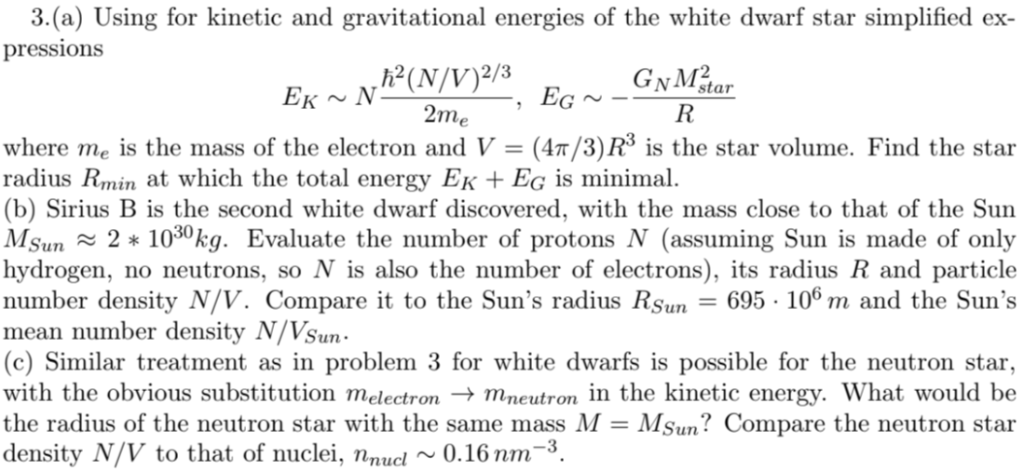3.(a) Using for kinetic and gravitational energies of the white dwarf star simplified ex pressions 2 NVI star 2me where me is the mass of the electron and V (4n/3) R3 is the star volume. Find the star radius Rmin at which the total energy Εκ + EC is minimal. (b) Sirius B is the second white dwarf discovered, with the mass close to that of the Sun Mun ะ 2 * 1030kg. Evaluate the number of protons N (assuming...

• ### 3 GM2 (5) The gravitational potential of a uniform-density sphere of mass M and radius R is E,-- Consider a white dwarf...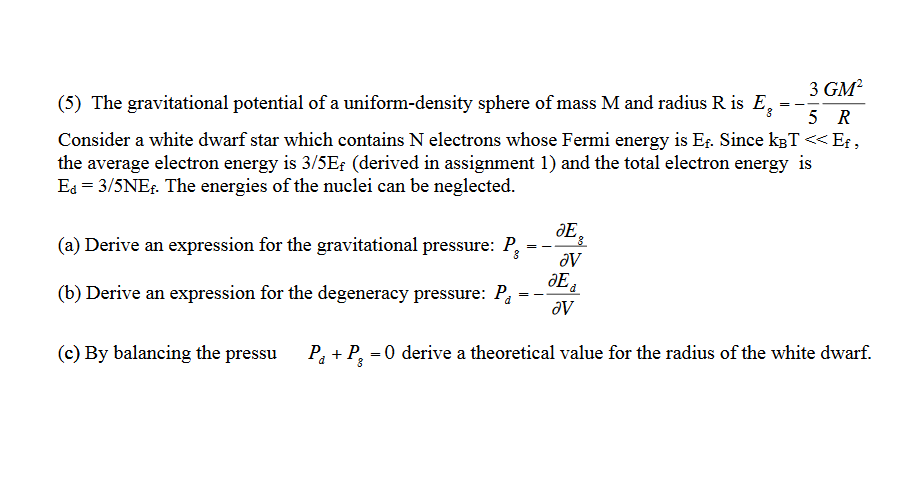3 GM2 (5) The gravitational potential of a uniform-density sphere of mass M and radius R is E,-- Consider a white dwarf star which contains N electrons whose Fermi energy is Es. Since kaT <<Ef, the average electron energy is 3/5Er (derived in assignment 1) and the total electron energy is Ed-3/5NEs. The energies of the nuclei can be neglected. OE (a) Derive an expression for the gravitational pressure: P- OE (b) Derive an expression for the degeneracy pressure: Pa-...

• ### Stars much heavier than our sun will not form white dwarf, but collapse further, becoming (if con...

Stars much heavier than our sun will not form white dwarf, but collapse further, becoming (if condition are right) neutron stars. They result from the supernova explosion of a massive star, combined with gravitational collapse, that compresses the core past white dwarf star density to that of atomic nuclei. Eventually neutron degeneracy pressure stabilizes the collapse, just as the electron does for white dwarfs. The Fermi Energy is given by where Z/A =1 and V corresponds to volume. The neutron...

• ### Imagine a hypothetical star of radius R, whose mass density ρ is constant throughout the star....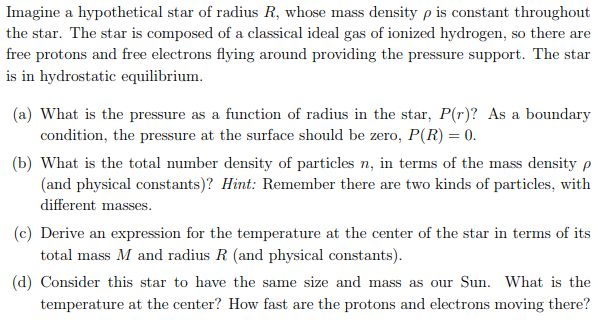Imagine a hypothetical star of radius R, whose mass density ρ is constant throughout the star. The star is composed of a classical ideal gas of ionized hydrogen, so there are free protons and free electrons flying around providing the pressure support. The star is in hydrostatic equilibrium (a) What is the pressure as a function of radius in the star, P(r)? As a boundary condition, the pressure at the surface should be zero, P(R) 0 (b) What is the...

• ### The star Tau Ceti's mass is 1.6 ✕ 1030 kg, its radius is 5.5 ✕ 105...

The star Tau Ceti's mass is 1.6 ✕ 1030 kg, its radius is 5.5 ✕ 105 km, and it has a rotational period of approximately 34 days. If Tau Ceti should collapse into a white dwarf of radius 7.5 ✕ 103 km, what would its period (in s) be if no mass were ejected and a sphere of uniform density can model Tau Ceti both before and after?

• ### 5) A star (no matter what its mass) spends most of its life Select one: a....

5) A star (no matter what its mass) spends most of its life Select one: a. as a protostar. b. as a main-sequence star. c. as a planetary nebula. d. as a red giant or supergiant. 6) What is the ultimate fate of an isolated white dwarf? Select one: a. It will cool down and become a cold black dwarf. b. As gravity overwhelms the electron degeneracy pressure, it will explode as a nova. c. As gravity overwhelms the electron...

• ### Consider a white dwarf with the same mass as our sun. What would its radius need...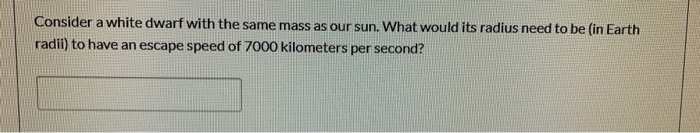Consider a white dwarf with the same mass as our sun. What would its radius need to be in Earth radii) to have an escape speed of 7000 kilometers per second?

• ### A neutron star has a radius of 10 km and a mass of 1.4 Solar masses,...

A neutron star has a radius of 10 km and a mass of 1.4 Solar masses, what is its density and how does this compare to the nuclear density of 2.3 x 1017 kg/m3 (One solar mass is 1.988 x 1030 kg)